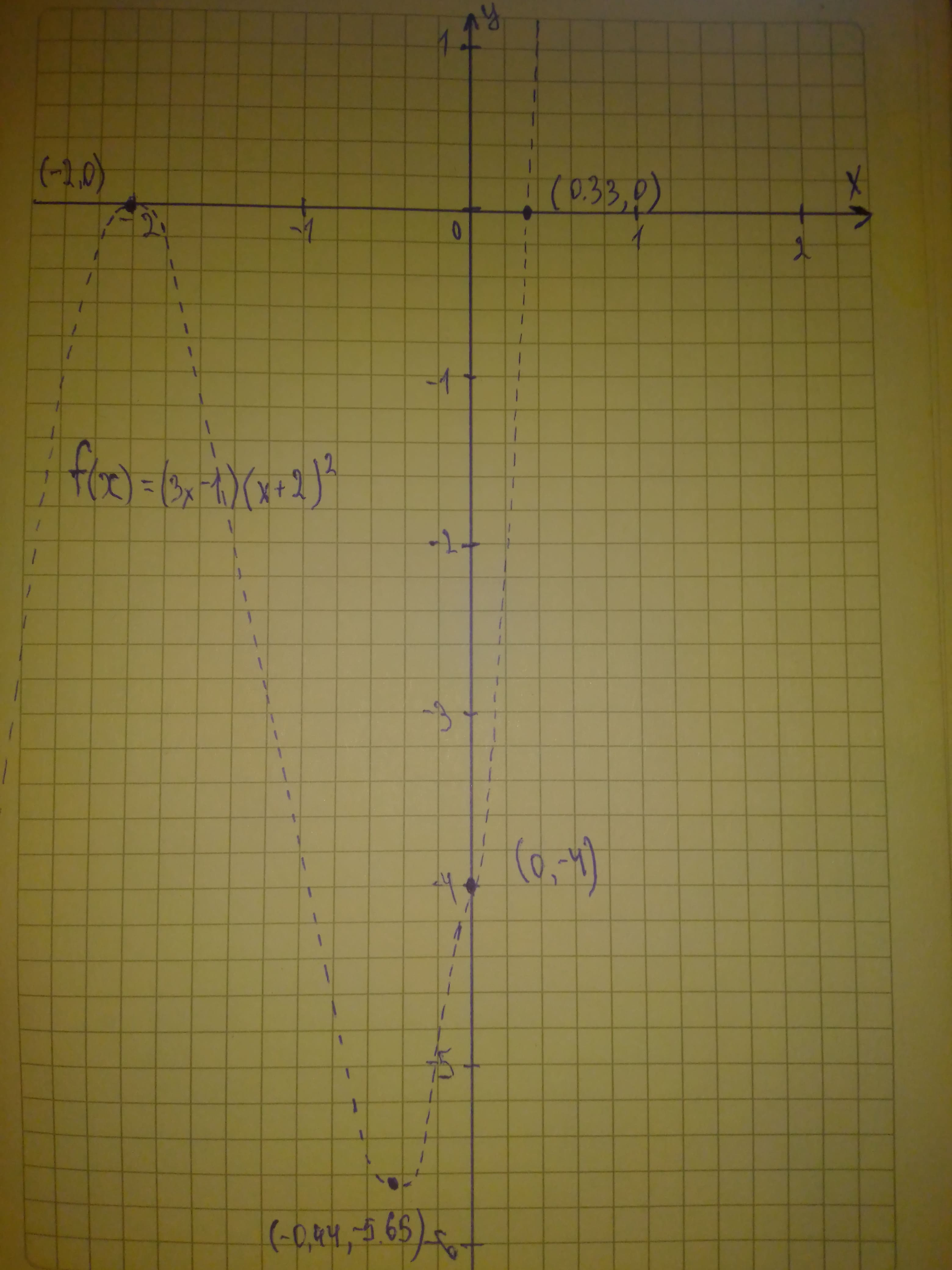Question# Graph each polynomial function. Factor first if the expression is not in factored form. f(x)=(3x-1)(x+2)^2

Polynomial graphs
ANSWEREDGraph each polynomial function. Factor first if the expression is not in factored form.
$$\displaystyle{f{{\left({x}\right)}}}={\left({3}{x}-{1}\right)}{\left({x}+{2}\right)}^{{2}}$$2021-06-132021-08-04

Step 1
$$\displaystyle{f{{\left({x}\right)}}}={\left({3}{x}-{1}\right)}{\left({x}+{2}\right)}^{{{2}}}$$ The function in factored form
The function has two zeros $$\displaystyle{\frac{{{1}}}{{{3}}}}$$ and -2
So, the graph of f(x) crosses the x-axis at $$\displaystyle{\left({\frac{{{1}}}{{{3}}}},{0}\right)}{\quad\text{and}\quad}{\left(-{2},{0}\right)}$$
To find the y-intercept, substitute 0 for x in f(x)
$$\displaystyle{f{{\left({x}\right)}}}={\left({3}{x}-{1}\right)}{\left({x}+{2}\right)}^{{{2}}}$$
$$\displaystyle{f{{\left({0}\right)}}}={\left({3}{\left({0}\right)}-{1}\right)}{\left({0}+{2}\right)}^{{{2}}}$$ Substitute 0 for x
$$\displaystyle={\left(-{1}\right)}{\left({2}\right)}^{{{2}}}$$ Simplify
$$\displaystyle=-{4}$$
So, the function f(x) crosses the y-axis at $$\displaystyle{\left({0},-{4}\right)}$$
Step 2
$$\displaystyle{3}{x}\cdot{\left({x}\right)}^{{{2}}}={3}{x}^{{{3}}}$$
$$\displaystyle{x}\rightarrow\infty,{f{{\left({x}\right)}}}\rightarrow\infty$$
$$\displaystyle{x}\rightarrow-\infty,{f{{\left({x}\right)}}}\rightarrow-\infty$$